# [Improving Deep Neural Networks] week2. Optimization algorithms

This week: optimization algos to faster train NN, on large dataset.

### batch v.s. mini-batch GD

Compute J on `m` examples: vectorization, i.e. stacking x(i) y(i) horizontally.
`X = [x(1), ..., x(m)]`
`Y = [y(1), ..., y(m)]`
→ still slow or impossible with large `m`.

⇒ split all m examples into mini-batches. X^t^, Y^t^
e.g. mini batch size = 1000.Minibatch GD:
each step, run one iteration of GD using X{t}, Y{t} instead of doing with full X, Y.
one "epoch": one pass through all training set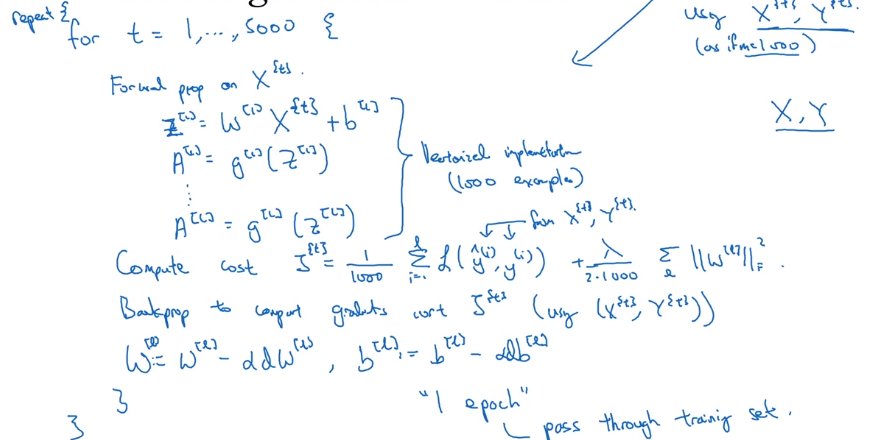with batch-GD: each iteration will decrease cost function.
in mini-batch: cost J^t^ is computed on different dataset — noisy.How to choose minibatch size

• extreme 1: minibatch size = m → batch GD

coverge fastest in each iter (but too long time or impossible per iter)

• extreme 2: minibatch size = 1 → stochastic GD

noisy, never coverge, (but loose all vectorization speedup)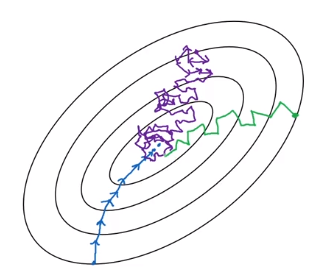Guidelines on choosing batch size:

• small training set (m<2000) → just batch size
• otherwise:

typical minibatch size = 64/128/256/512 (make sure minibatch size fits in CPU/GPU memory)

## Exponentially weighted (moveing) averages

In fact, exp-weighted-avg is an non-parametric estimator/smoother of a series of vlaues.

example: Temperature over the year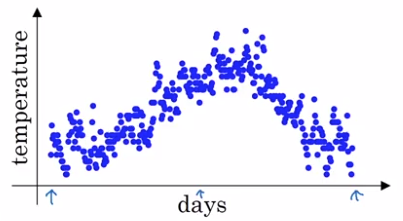→ use a exp-weighted moving average to model this:
`theta[t]` = temperature at day t, t = 1,2,3,...
`v[t]` = averaged(smoothed) estimate of theta, t = 0,1,2,3,...
exp-weighted average: recursivly compute v[t].
`v = 0, v[t] = 0.9 * v[t-1] + 0.1 * theta[t]`
(param: beta = 0.9)
⇒ v[t] ~= average of theta[t] over the last 1/(1-beta) days. (c.f. next section)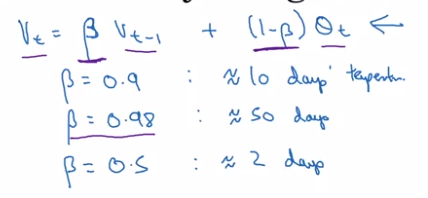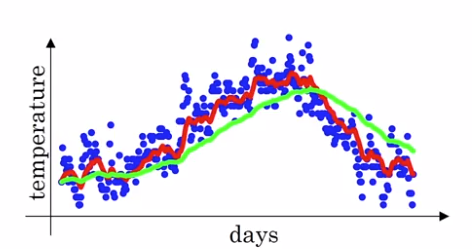## Understanding exponentially weighted averagesUnderstanding the math of exp-weighted-average:
→ unroll the recursive formula:⇔ v = a convolution of theta[t-100:t] and a exp-decaying function: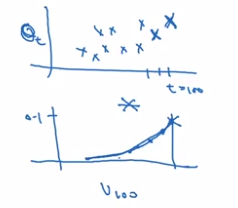A small trick on estimating exps: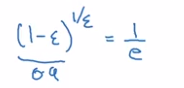That's why in previous section, we say this formula ~= averaging over last 1/(1-beta) (=1/epslon) days' theta.
e.g. theta[t-10]'s weight is 0.9 ^ 10 ~= 1/e ~= 0.35, i.e. after 10 days, the weight of theta[t-10] is decayed to ~< 1/3.

Advantage to just computing moving window averages: in implementation, only keep updating a single variable v_theta — very small memory usage.

## Bias correction in exponentially weighted averages

To make exp-weighted averages more accurate by bias correction.
Problem with previous implementation in initial phase:

• v = 0, beta = 0.98
• v = 0.980 + 0.02 * theta ⇒ v[t] starts lower than theta. *(purple curve VS green curve)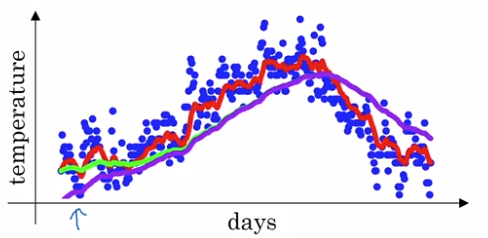Correction:
take `v[t] / (1 - beta^t)` instead of v[t].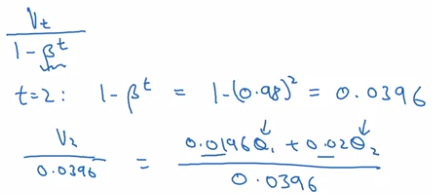In practice: most people don't bother to implement bias correction — just wait for the initial phase to warm up...

GD with momentum:

• almost always faster than normal GD
• in short: compute exp-weighted-avg of the gradients as gradient to use

example: if contour of loss is an ellipse, can't use too large step in GD, and oscillate. → average of steps will be faster.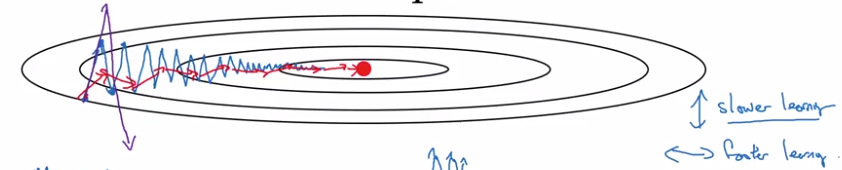GD momentum:
Use exp-weighted-avg of dW (V_dW) and of db (V_db). — smooth out oscillation steps of normal GD.
update params with the averaged value V_dW, V_db.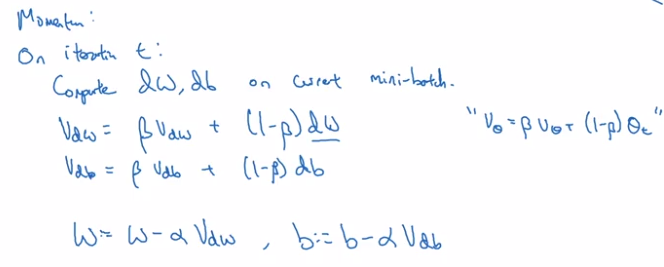Why the name "momentum": rolling down a ball in a bowl
dW/db: ~accelation
V_dW/B_db: ~velocity at current time (this is why the smoothing average is called 'v')
beta: ~friction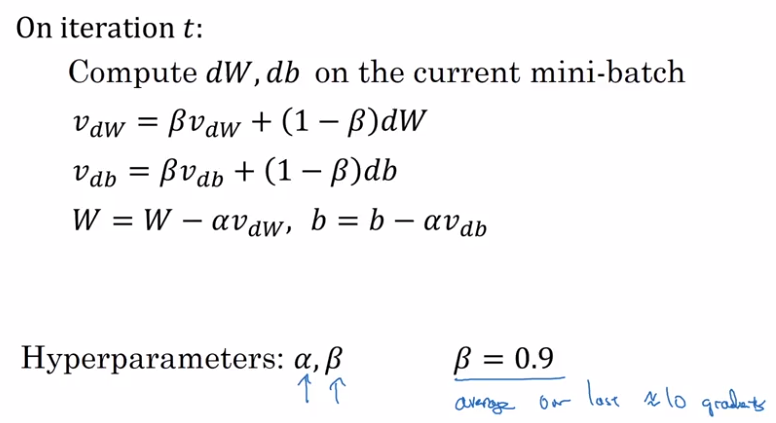in practice:

• beta=0.9 works well for most cases
• no bias correction implemented
• can use beta = 0 → ~V_dW is scaled by 1/(1-beta) → use a scaled alpha then.

⇒ less intuitive, and couples the tuning of alpha and beta

## RMSprop

"Root-Mean-Square-prop".

• also for speedup GD
• in short:

S = exp-weighted-avg of gradient squared (that's why call param beta2);
when updating params(W,b), scale dW,db by sqrt of S. `dW / sqrt(S_dW)`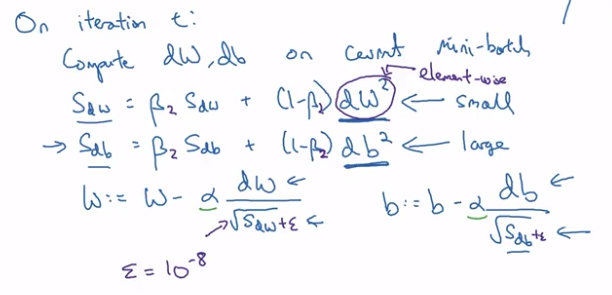example: ellipse contour, want slow rate in `b` directrion and fast rate in `w` direction.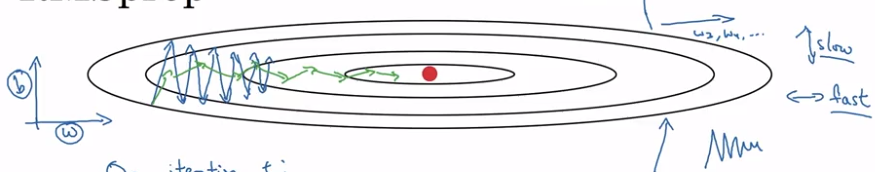⇒ for each step (dW, db) = gradient of current minibatch:

• db large, dW small

→ update w by dW/sqrt(S_dW), ⇔ larger step in W direction
→ db/sqrt(S_db) ⇔ smaller step in b direction → damping out oscillations in b direction

• A combination of RMSprop and momentum.
• Proved to work well for a varity of problems.

Algo:

• maintain both V_dW, V_db (hyper-param=beta1) and S_dW, S_db (hyper-param=beta2)
• implement bias correction: V_corrected, S_corrected — divid by (1-beta^t)
• param update (W, b): `V / sqrt(S)`hyperparameters:

• alpha: learning rate, needs tuning
• beta1: usually 0.9
• beta2: usually 0.999
• epsilon: 10e-8 (not important)

## Learning rate decay

slowly reduce learning rate.

In minibatch with fixed learning rate: will never converge.
⇒ decay learning rate → oscillating around a smaller region of optima.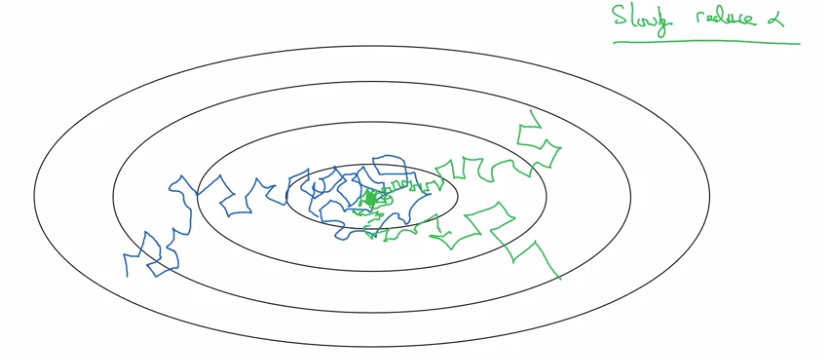Implementation:

• 1 epoch = 1 pass through whole data.
• decay learning rate alpha after each epoch (hyper-param decay-rate):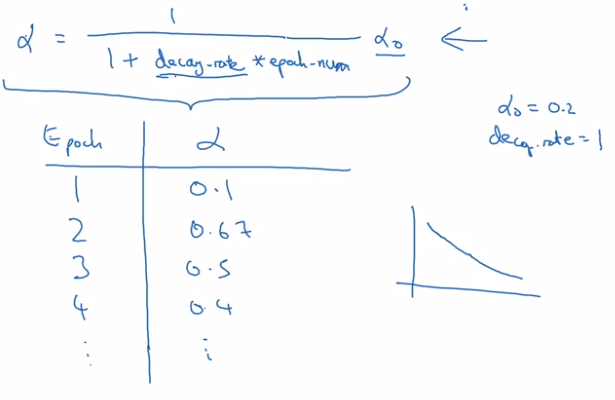other decay method:

• exponentially decay alpha:• sqrt of epoch_num:• discrete staircase: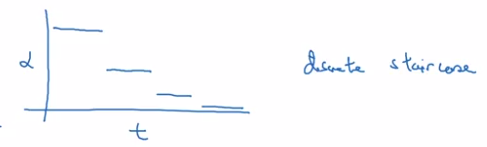• manual decay: when training takes long time

## The problem of local optima

low-dimention optimas: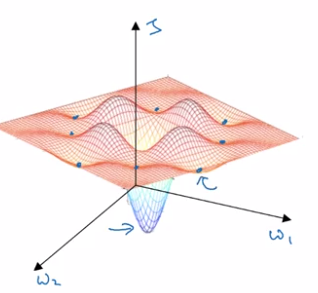This gives wrong intuition, in practice (high-dim), most 0-gradient points are saddle points.plateau: region where gradient close to 0 for long time.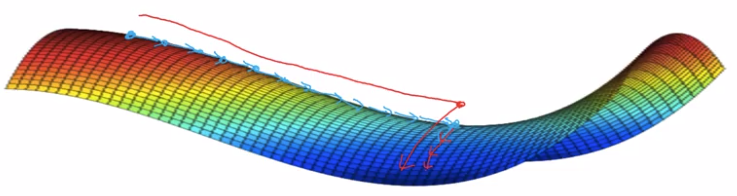take-away:

• unlikely to stuck in bad local optima: D dimentional → ~2^(-D) of chance.
• plateaus can make learning slow → use momentum/RMSprop/Adam to speedup training.

## assignment

implementing mini-batch GD:
shuffle data (note: X and Y's columns are sync-ed!):

```permutation = list(np.random.permutation(m))
shuffled_X = X[:, permutation]
shuffled_Y = Y[:, permutation].reshape((1,m))
```

→ partition data:
kth mini batch:

```mini_batch_X = shuffled_X[:, k*mini_batch_size:(k+1)*mini_batch_size]
mini_batch_Y = shuffled_Y[:, k*mini_batch_size:(k+1)*mini_batch_size]
```

detail: when m % minibatch_size != 0: handle last batch (smaller than a regular batch)